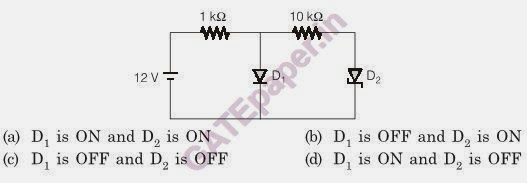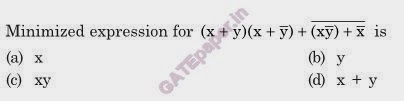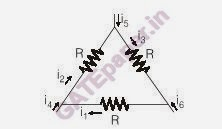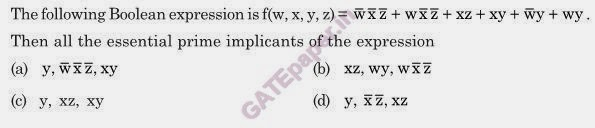### GATE 2014 Video Solutions on EDC, Analog, Digital and Signals & Systems

1. In the voltage regulator shown, OP-AMP is ideal, and the BJT has VBE = 0.7volts and ß = 100, then find the value of R in ohms.2. A depletion type N-channel MOS is biased in its linear region to use as a voltage controlled resistor. Assume Vth = 0.5 volts, VGS = 20 volts, VDS = 5 volts, W/L = 100, COX = 10-8 F/m2, µn = 800 cm2/V-sec. Find teh resistance of voltage controlled resistor in ohms.

3.  In BJT transistor VBE = 0.7 volts, VT = 25 mV, and reverse saturation current is 10-13 Amp. Find the transconductance in mA/V.

4. Find the RMS value of the given Pulse5. Find ROC of  Z-Transform of the given signal6. For the circuit given below, what will be the largest value of arm, when it is converted into delta form.7.  When the optical power incident on photo diode is 10 µW and the respponsitivity R = 0.8 A/W, then the photo current generated is ........................ (in µA)

8. The value of output F of the given circuit is9. In the figure, there is a low pass filter with a cut-off frequency of 5 KHz. The value of R2 in KOhms is10. In the figure shown, has a PN junction with a cutoff voltage of 0.7 volts, and a schottky diode with a cut-off votlaage of 0.3 volts. If ON indicates conducting state and OFF indicates non-conducting state then,11. Find the fundamental period of the signal x[n]12. Ideal current buffer is having
(a)  Low input impedance and high output impdeance
(b)  High input impedance and high output impdeance
(c)  High input impedance and low output impdeance
(d)  Low input impedance and low output impdeance

13.  Minimized expression for the given expression is14. For the given circuit, where R = 1Ohm, i1 = 2A, i4 = -1A, i5 = -4A. Then which of the following is correct.(a) i6 = 5 A
(b) i3 = -4 A
(c) Given data sufficient to tell these currents are not possible
(d) Data is non sufficient to find i2, i3 and i5

15. For the given Boolean expression, find all the essential prime implicants of the expression.16. In the given circuit, if A is connected to Q1, the operation of the circuit is according to the state diagram. If XOR is replaced with XNOR, then to get the same operation of the circuit, which of the following changes has to be done.17. For the given circuit, the output voltage Vo is18. Find the voltage observed at P, Q and R for NMOS transistor and threshold voltage of 1 volt.19. The input frequency for the given counter is 1MHz, then the output frequency observes at Q4 is..........20.  For the circuit given, if the clock frequency is 1 KHz, then the frequency of output at Q3 is .......